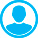FRACTAL CHARACTERISTICS OF SEISMIC PROCESS IN ROCK MASS AT MINING: MATHEMATICAL MODELING AND ANALYSISAbstract (English):
It is shown in the paper that the system of equations of solid mechanics, which has a mixed type, demonstrate the most common features of evolution of nonlinear dynamic systems. Previous investigations of seismic process were carried out on the base of simplified (sand-pile, land-slide) models which gave a graph of recurrence of seismic events and information about the state of self-organized criticality (SOC). However, these simplified models do not contain the information about the stress-strain state of the loaded geomedia and its proximity to the critical state. In the proposed paper the model of rock mass with excavation is constructed and general step of roof caving is modelled. On the base of these modeling the formation of critical state in loaded geomedia is studied. The fluctuations of stress-strain state at different points of geomedia are studied as the reflection of fracture process occurring in the main elements of rock mass: roof and floor, when the coal face is advanced. It is shown that the probability distribution function (PDF) dependencies, amplitude-frequency characteristics reflect the state of the rock mass and might be considered as the fractal characteristics of fracture process within. The evolution of these dependencies shows the dramatic change when the critical state is formed in the rock mass surrounding the underground opening.

Keywords:
Mathematical modeling, fractal characteristics, coal mining, roof caving, stress fluctuations, critical states, damage accumulation

1. Chanyshev, A. I., Abdulin, I. M. Stress-strain state of enclosing rock mass around an arbitrary cross-section excavation by measurement of displacements of the excavation walls, // Journal of Mining Science, 2014. - v. 50 - p. 18.

2. Demin, V. F., Nemova, N. A., Demina, T. V. Analytical modeling of geomechanical processes in the marginal array mining, // Journal of Siberian Federal University. Engineering and Technologies, 2015. - v. 1 - p. 74.

3. Eremin, M. O., et al. Modelling of processes of damage accumulation and multiscale fracture in rock mass with excavations at mining // AIP Conference proceedings 1683, 020048-1-4 - New York: AIP Publishing., 2015. - p. 1.

4. Khoshboresh, A. R. Memoire - Canada: University LAVAL., 2013. - 155 pp.

5. Kiya, O. E., Hosseinitoudeshki, V. The three dimensional strain in cylindrical tunnels, // WALIA Journal, 2015. - v. 31 - p. 172.

6. Kwasniewski, M. Numerical analysis of strata behavior in the vicinity of a longwall panel in a coal seam mined with roof caving // Continuum and Distinct Element Numerical Modelling in Geo-Engineering, Paper: 07-08 - Minneapollis: Itasca., 2008. - p. 1.

7. Lu, Y., Yang, W. Analytical solutions of stress and displacement in strain softening rock mass around a newly formed cavity, // Journal of Central South University, 2013. - v. 20 - p. 1397.

8. Makarov, P. Mathematical theory of evolution of loaded solids and media, // Physical Mesomechanics, 2008. - v. 11 - p. 19.

9. Makarov, P. V., Eremin, M. O., Kostandov, Yu. A. Mathematical theory of evolution of loaded solids and media. Physical mesomechanics, // Physical Mesomechanics, 2014. - v. 17 - p. 199.

10. Nuric, A., Nuric, S., Kricak, L., Lapandic, I., Husagic, R. Numerical modeling and computer simulation of ground movement above underground mine, // World Academy of Science, Engineering and Technology, 2012. - v. 6 - p. 316.

11. Pardoen, B., Collin, F., Levasseur, S., Charlier, R. Modelling the excavation damaged zone in Callovo-Oxfordian claystone with strain localization // ALERT Workshop - Aussois: Univerite de Liege Publishing., 2012. - p. 1.

12. Pavlova, L. D., Fryanov, V. N. Modelling of cyclic collapse of rocks at mining with sequential damage accumulation, // Izvestiya Tomskogo Politechnicheskogo Universiteta, 2004. - v. 307 - p. 76.

13. Roatesi, S. Analysis of the successive phases of a tunnel excavation with support mounting, // Proceedings of the Romanian academy, Series A, 2010. - v. 11 - p. 11.

14. Shabanimashcool, M. Numerical modeling of the longwall mining and the stress state in Svea Nord Coal Mine - Trondheim, Norway: Norwegian University of Science and Technology., 2012.

15. Shapoval, A. B., Sniirman, M. G. Universality of algorithmic prognosis of extremal events in time series, // Information Technologies and Calculation Systems, 2011. - v. 4 - p. 58.

16. Shebalin, P., Keilis-Borok, V. I., Gabrielov, A., Zaliapin, L., Turcotte, D. Short-term earthquake prediction by reverse analysis of lithosphere dynamics, // Tectonophysics, 2006. - v. 413 - p. 63.

17. Verma, A. K., Deb, D. Numerical analysis of interaction between hydraulic-powered support and surrounding rock strata, // International Journal of Geomechanics, 2013. - v. 13 - p. 181.

18. Wang, G., Wang, H. Y., Huang, Q. M., Su, C. Q. Numerical modeling of rock cross-cut coal uncovering based on ANSYS, // Computer Modelling and New Technologies, 2014. - v. 18 - p. 26.

19. Yang, H. Q., Zeng, Y. Y., Lan, Y. F., Zhou, X. P. Analysis of the excavation damaged zone around a tunnel accounting for geostress and unloading, // International Journal of Rock Mechanics and Mining Sciences, 2014. - v. 69 - p. 59.

20. Zhu, W. C., Liu, J., Tang, C. A., Zhao, X. D., Brady, B. H. Simulation of progressive fracturing processes around underground excavations under biaxial compression, // Tunneling and Underground Techn., 2005. - v. 20 - p. 231.